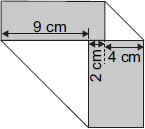# User ForumSubject :IMO    Class : Class 5

Two corners of a napkin are folded as shown. Find the area of the napkin when it is unfolded.A 60 cm2
B 225 cm2
C 195 cm2
D 450 cm2

## Ans 1:

Class : Class 8
Thank-you for giving us the solution

## Ans 2:

Class : Class 6
thanks. I didn't get the answer at first

## Ans 3:

Class : Class 5
I forgot to attempt this question but when I saw this, it appeared a bit difficult to me, but after calculating, I was sure that the answer is C.

Class : Class 5

## Ans 5:

Class : Class 5
I thought that it's in a square shape...â¦â¦â¦.that is why I did 15 X 15 not 15 X 13....

## Ans 6:

Class : Class 5
Answer: C Explanation: Add all the numbers given their to find the length. It is 9+2+4=15 Unfold the napkin. you will get a number 9 at the left hand side with some space left. you will get a number 4 on the right hand side with some space left. if you imagine this or look at this carefully, you will notice that the space left at the either sides is actually 4 and 9 respctively. so, to find the breadth, add thesess no.-- 9+4=13. So l=151, b=13. Area is being asked. Formula of calculating area of a rectangle: lXb= 15 X 13 = 195 cm'2 Hence, option C is correct.

## Ans 7:

Class : Class 5
length is 15 and breadth is 13. There was a silly mistake in it.

Class : Class 8

## Ans 9:

Class : Class 5
thANKS FOOR SOLUTION

Class : Class 6

Class : Class 7

Class : Class 8

## Ans 13:

Class : Class 7
C is the answer to this question

## Ans 14:

Class : Class 6
Thank you for the solution :)

## Ans 15:

Class : Class 6
Answer should be Option B because in the solution the napkin unfolded looks like a square and a squareâs all sides are equal so itâs area should be = 15*15= 225 sq.cm .